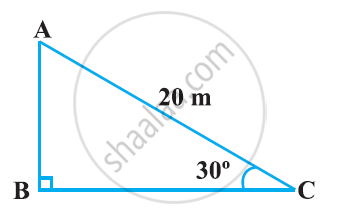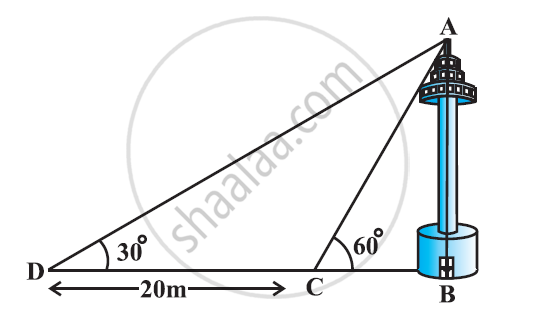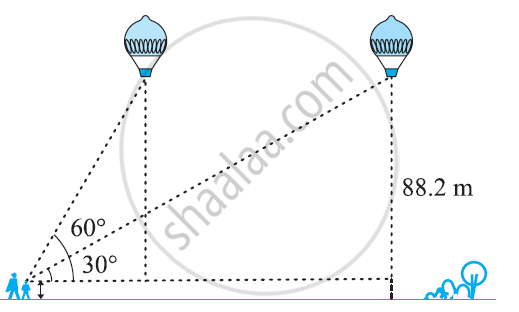# NCERT solutions for Class 10 Maths chapter 9 - Some Applications of Trigonometry [Latest edition]

#### Chapters## Solutions for Chapter 9: Some Applications of Trigonometry

Below listed, you can find solutions for Chapter 9 of CBSE NCERT for Class 10 Maths.

Exercise 9.1
Exercise 9.1 [Pages 203 - 205]

### NCERT solutions for Class 10 Maths Chapter 9 Some Applications of Trigonometry Exercise 9.1 [Pages 203 - 205]

Exercise 9.1 | Q 1 | Page 203

A circus artist is climbing a 20 m long rope, which is tightly stretched and tied from the top of a vertical pole to the ground. Find the height of the pole, if the angle made by the rope with the ground level is 30 °Exercise 9.1 | Q 2 | Page 203

A tree breaks due to storm and the broken part bends so that the top of the tree touches the ground making an angle 30 ° with it. The distance between the foot of the tree to the point where the top touches the ground is 8 m. Find the height of the tree.

Exercise 9.1 | Q 3 | Page 203

A contractor plans to install two slides for the children to play in a park. For the children below the age of 5 years, she prefers to have a slide whose top is at a height of 1.5 m, and is inclined at an angle of 30 ° to the ground, where as for the elder children she wants to have a steep side at a height of 3 m, and inclined at an angle of 60 ° to the ground. What should be the length of the slide in each case?

Exercise 9.1 | Q 4 | Page 204

The angle of elevation of the top of a tower from a point on the ground, which is 30 m away from the foot of the tower, is 30°. Find the height of the tower

Exercise 9.1 | Q 5 | Page 204

A kite is flying at a height of 60 m above the ground. The string attached to the kite is temporarily tied to a point on the ground. The inclination of the string with the ground is 60°. Find the length of the string, assuming that there is no slack in the string.

Exercise 9.1 | Q 6 | Page 204

A 1.5 m tall boy is standing at some distance from a 30 m tall building. The angle of elevation from his eyes to the top of the building increases from 30° to 60° as he walks towards the building. Find the distance he walked towards the building

Exercise 9.1 | Q 7 | Page 204

From a point on the ground, the angles of elevation of the bottom and the top of a transmission tower fixed at the top of a 20 m high building are 45° and 60° respectively. Find the height of the tower. (Use sqrt3 = 1.73)

Exercise 9.1 | Q 8 | Page 204

A statue, 1.6 m tall, stands on a top of pedestal, from a point on the ground, the angle of elevation of the top of statue is 60° and from the same point the angle of elevation of the top of the pedestal is 45 °. Find the height of the pedestal.

Exercise 9.1 | Q 9 | Page 204

The angle of elevation of the top of a building from the foot of the tower is 30° and the angle of elevation of the top of the tower from the foot of the building is 60°. If the tower is 50 m high, find the height of the building

Exercise 9.1 | Q 10 | Page 204

Two poles of equal heights are standing opposite each other an either side of the road, which is 80 m wide. From a point between them on the road, the angles of elevation of the top of the poles are 60° and 30º, respectively. Find the height of poles and the distance of the point from the poles.

Exercise 9.1 | Q 11 | Page 204

A TV tower stands vertically on a bank of a canal. From a point on the other bank directly opposite the tower the angle of elevation of the top of the tower is 60°. From another point 20 m away from this point on the line joining this point to the foot of the tower, the angle of elevation of the top of the tower is 30°. Find the height of the tower and the with of the canal.Exercise 9.1 | Q 12 | Page 204

From the top of a 7 m high building, the angle of elevation of the top of a cable tower is 60° and the angle of depression of its foot is 45°. Determine the height of the tower.

Exercise 9.1 | Q 13 | Page 204

As observed from the top of a 75 m high lighthouse from the sea-level, the angles of depression of two ships are 30° and 45°. If one ship is exactly behind the other on the same side of the lighthouse, find the distance between the two ships.

Exercise 9.1 | Q 14 | Page 205

A 1.2 m tall girl spots a balloon moving with the wind in a horizontal line at a height of 88.2 m from the ground. The angle of elevation of the balloon from the eyes of the girl at any instant is 60°. After some time, the angle of elevation reduces to 30°. Find the distance travelled by the balloon during the interval.Exercise 9.1 | Q 15 | Page 205

A straight highway leads to the foot of a tower. A man standing at the top of the tower observes a car as an angle of depression of 30°, which is approaching the foot of the tower with a uniform speed. Six seconds later, the angle of depression of the car is found to be 60°. Find the time taken by the car to reach the foot of the tower from this point.

Exercise 9.1 | Q 16 | Page 205

The angles of elevation of the top of a tower from two points at a distance of 4 m and 9 m. from the base of the tower and in the same straight line with it are complementary. Prove that the height of the tower is 6 m.

## Solutions for Chapter 9: Some Applications of Trigonometry

Exercise 9.1## NCERT solutions for Class 10 Maths chapter 9 - Some Applications of Trigonometry

Shaalaa.com has the CBSE Mathematics Class 10 Maths CBSE solutions in a manner that help students grasp basic concepts better and faster. The detailed, step-by-step solutions will help you understand the concepts better and clarify any confusion. NCERT solutions for Mathematics Class 10 Maths CBSE 9 (Some Applications of Trigonometry) include all questions with answers and detailed explanations. This will clear students' doubts about questions and improve their application skills while preparing for board exams.

Further, we at Shaalaa.com provide such solutions so students can prepare for written exams. NCERT textbook solutions can be a core help for self-study and provide excellent self-help guidance for students.

Concepts covered in Class 10 Maths chapter 9 Some Applications of Trigonometry are Heights and Distances, Heights and Distances.

Using NCERT Class 10 Maths solutions Some Applications of Trigonometry exercise by students is an easy way to prepare for the exams, as they involve solutions arranged chapter-wise and also page-wise. The questions involved in NCERT Solutions are essential questions that can be asked in the final exam. Maximum CBSE Class 10 Maths students prefer NCERT Textbook Solutions to score more in exams.

Get the free view of Chapter 9, Some Applications of Trigonometry Class 10 Maths additional questions for Mathematics Class 10 Maths CBSE, and you can use Shaalaa.com to keep it handy for your exam preparation.

Share